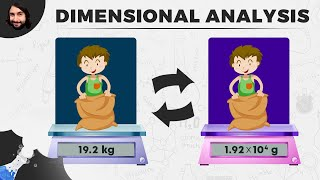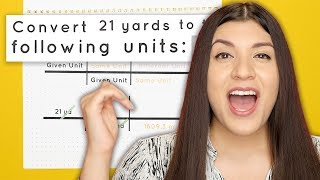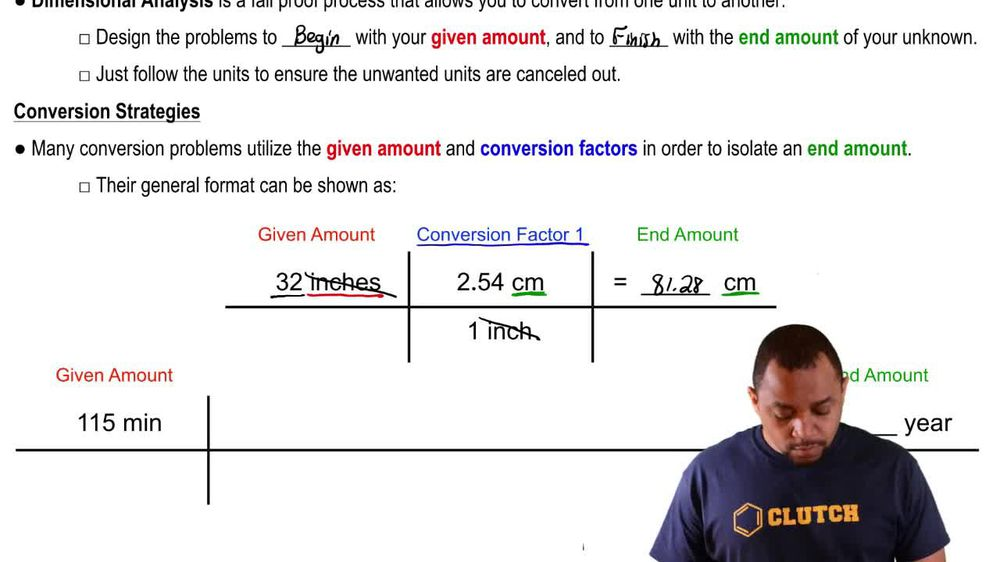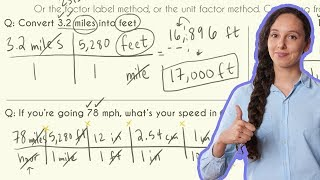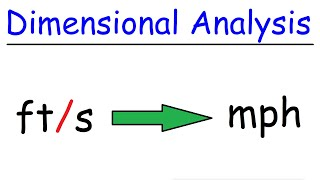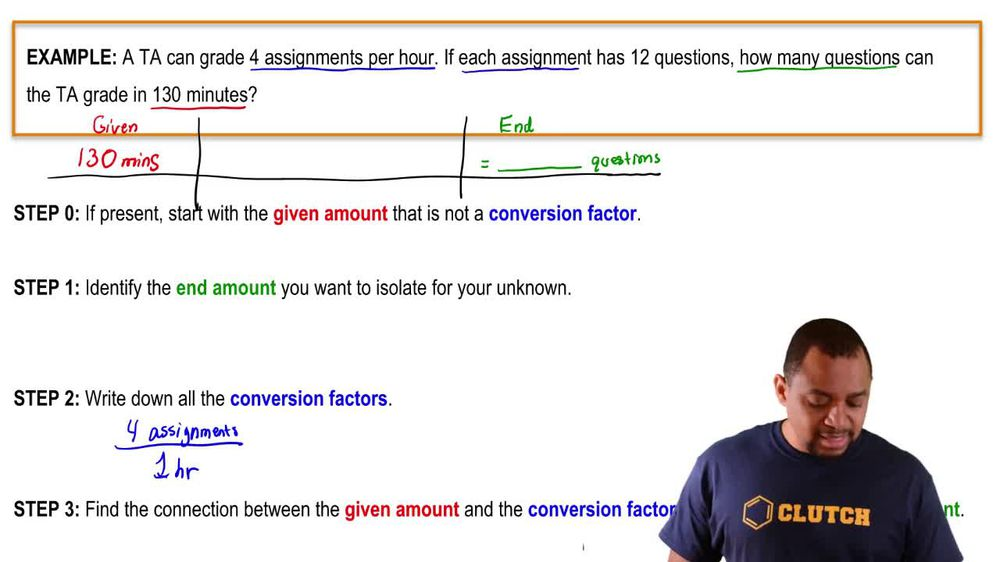Start typing, then use the up and down arrows to select an option from the list.
1. 1. Intro to General Chemistry2. Dimensional Analysis
Problem

# The height of a horse is usually measured in hands instead of in feet, where 1 hand equals 1/3 ft (exactly). (b) What is the volume in cubic meters of a box measuring 6 * 2.5 * 15 hands

Relevant Solution2m
Play a video:
Hey everyone, we're told if we express a cat's height and finger measurements rather than feet where one ft equals 2.67 fingers, what is the volume and cubic inches of a three by 2.8 by 1. fingers, rectangular prism first. Let's go ahead and determine the volume of our rectangular prism. Now we know our volume will be equal to r length times are with times our height. So plugging in these values, we get 3 2.8 times, 1.2 Which gets us to a volume of 10.08 cubic fingers. Since each of our values had a unit of fingers. Now, we want to go ahead and use our dimensional analysis here and convert our 10.08 cubic fingers into cubic inches. First we want to convert our cubic fingers into cubic feet. So we were told that we had 2.67 fingers per one ft. And since we are in cubic fingers we have to cube each of these values. Next we know that per one ft We will have 12". And again, since we do want our answer to be in cubic inches, we will cube each of these values in order to get that final unit. So when we calculate this out and cancel out our units, we end up with a total of 915 cubic inches, Which we can further simplify into nine times 10 squared cubic inches. Now, I hope that made sense and let us know if you have any questions